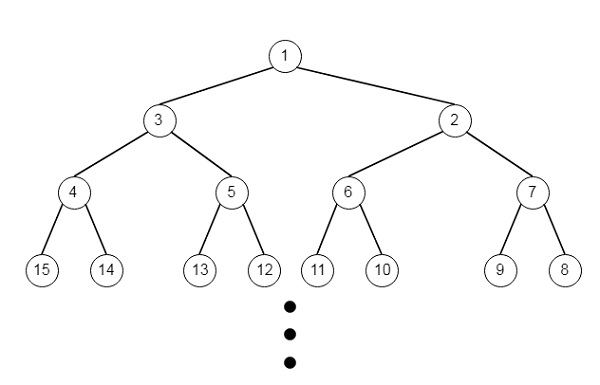# Path In Zigzag Labelled Binary Tree in Python

Suppose in an infinite binary tree where every node has two children, the nodes are labelled in row order. Now in the odd numbered rows (the first, third, fifth,...), the labelling is left to right, and in the even numbered rows (second, fourth, sixth,...), the labelling is right to left. So the tree will be like −So we have given the label of a node in this tree, we have to find the labels in the path from the root of the tree to the node with that label. So if the input is label = 14, then the output will be [1,3,4,14]

To solve this, we will follow these steps −

• Define two array tree and res, insert 0 and 1 into the tree array, set odd := 1 and current := 1 and two := 2

• if label = 1, then return a list with single element 1.

• Create one infinite loop −

• if odd is non-zero, then

• max_val := current + two

• temp := max_val

• while temp > current

• insert temp into tree

• if temp = label, then come out from the loop

• decrease temp by 1

• if last element of tree is label, then come out from the loop

• current := max_val

• otherwise

• temp := two

• while temp is not zero

• temp := 1, increase current by 1, then increase current into tree

• if current = label, then come out form the loop

• if last element of tree is label, then come out from the loop

• temp := temp * 2

• odd := false if odd is non zero, otherwise true

• index := length of tree – 1

• while index is not 0

• insert tree[index] into res array

• index := index / 2

• res := reversed list of res

• return res

## Example(Python)

Let us see the following implementation to get a better understanding −

Live Demo

class Solution(object):
def pathInZigZagTree(self, label):
tree = []
res = []
tree.append(0)
tree.append(1)
odd = 1
current = 1
two = 2
if label == 1:
return 
while True:
if odd:
max_val = current + two
temp = max_val
while temp>current:
tree.append(temp)
if temp == label:
break
temp-=1
if tree[-1]== label:
break
current = max_val
else:
temp = two
while temp:
temp-=1
current+=1
tree.append(current)
if current == label:
break
if tree[-1]== label:
break
two*=2
odd = not odd
index = len(tree)-1
while index:
res.append(tree[index])
index//=2
res=res[::-1]
return res
ob = Solution()
print(ob.pathInZigZagTree(14))

## Input

14

## Output

[1,3,4,14]